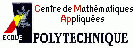Consulting for the Physics Department (Polytchnique)In the calculation of absorption spectra one needs to compute an eigenvalue decomposition of a hermitian Hamiltonian which consists of the sum of two matrices: A dense hermitian matrix with known eigenvalues and eigenvectors and a structured hermitian matrix. The structured matrix represents a short range interaction and contains only elements in a small block in the upper left corner. To compute the eigenvalue decomposition I suggested
and implemented a prototype updating algorithm which can be found in the following report: During the consulting, it turned out that the eigenvalue decomposition was not really
needed. The real problem was to solve linear systems of equations with the hermitian
Hamiltonian. Thus Gene Golub suggested a different approach which does not require the
eigenvalue decomposition of the matrix any more: One can use the Sherman-Morrison-Woodbury formula to invert low rank pertrurbed large matrices for which the original inverse is known, which is here the case, since the original eigenvalue decomposition is known. I implemented a prototype of this algorithm which gives very promising results. The real implementation on the Cray thus considered.

[Home] [Teaching] [Research] [Consulting] [MathHome] [McGill]